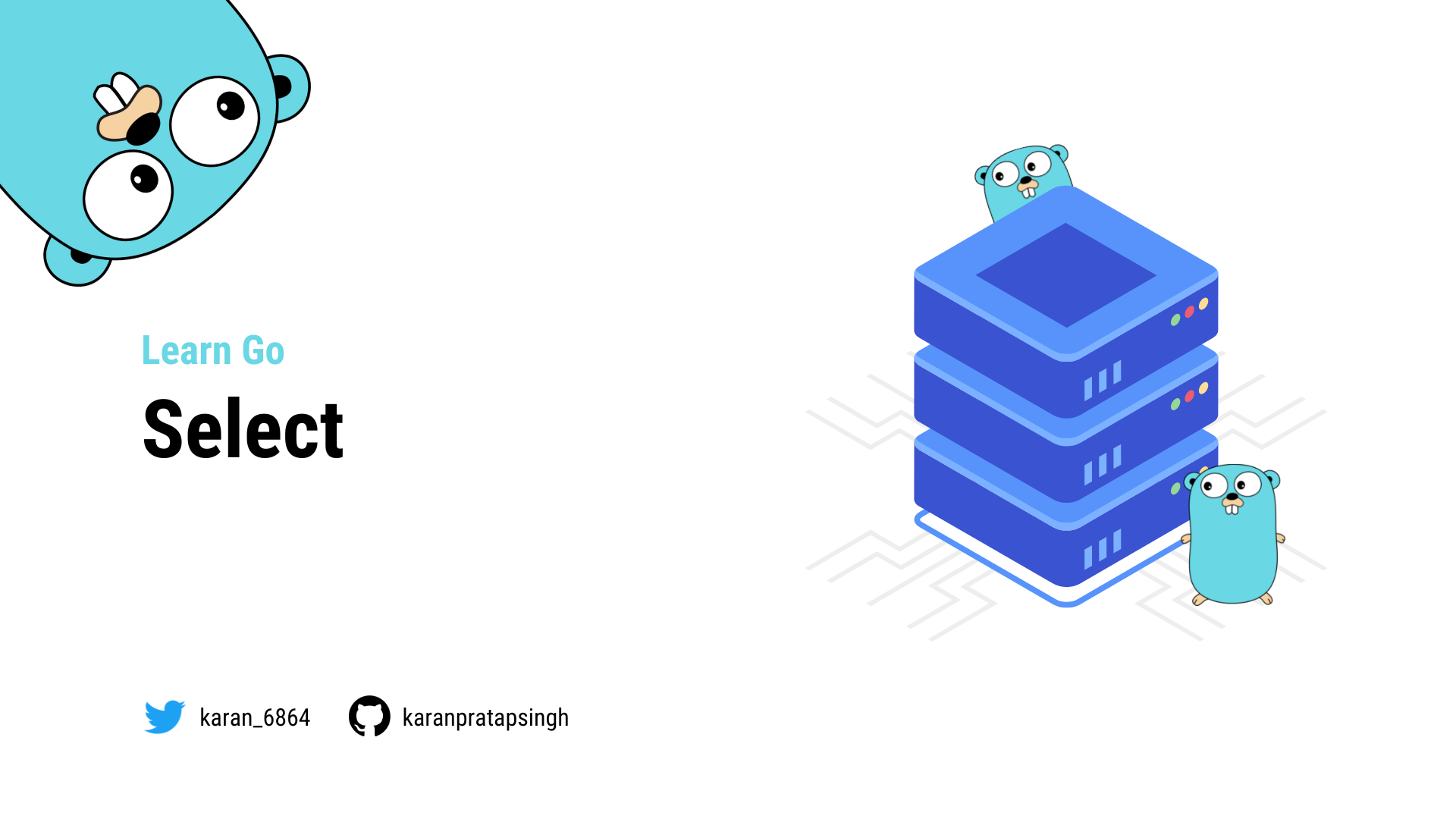# Select

Authors
•Name
Karan Pratap Singh
@karan_6864In this tutorial, we will learn about the `select` statement in Go.

The `select` statement blocks the code and waits for multiple channel operations simultaneously.

A `select` blocks until one of its cases can run, then it executes that case. It chooses one at random if multiple are ready.

``````package main

import (
"fmt"
"time"
)

func main() {
one := make(chan string)
two := make(chan string)

go func() {
time.Sleep(time.Second * 2)
one <- "One"
}()

go func() {
time.Sleep(time.Second * 1)
two <- "Two"
}()

select {
case result := <-one:
case result := <-two:
}

close(one)
close(two)
}
``````

Similar to `switch`, `select` also has a default case that runs if no other case is ready. This will help us send or receive without blocking.

``````func main() {
one := make(chan string)
two := make(chan string)

for x := 0; x < 10; x++ {
go func() {
time.Sleep(time.Second * 2)
one <- "One"
}()

go func() {
time.Sleep(time.Second * 1)
two <- "Two"
}()
}

for x := 0; x < 10; x++ {
select {
case result := <-one:
case result := <-two:
default:
fmt.Println("Default...")
time.Sleep(200 * time.Millisecond)
}
}

close(one)
close(two)
}
``````

It's also important to know that an empty `select {}` blocks forever.

``````func main() {
...
select {}

close(one)
close(two)
}
``````